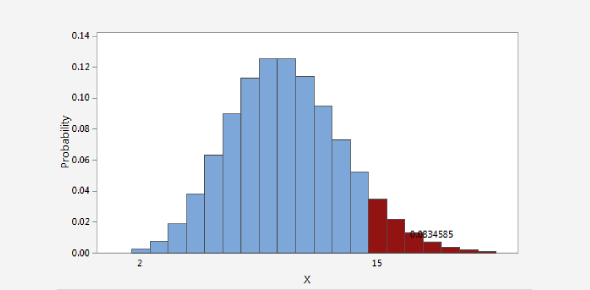# Probability Distribution: MCQ Quiz!

8 Questions | Total Attempts: 100Settings.

• 1.
In binomial probability distribution, dependents of standard deviations must include:
• A.

Probability of q

• B.

Probability of p

• C.

Trials

• D.

All of above

• 2.
If event A and B are independent then P(A/B)
• A.

P(A)

• B.

P(A intersection B)

• C.

P(B)

• D.

P(A). P(B)

• 3.
The odds in favor of drawing a king or a diamond from a well-sheffled pack of 52 cards are?
• A.

9:4

• B.

4:9

• C.

5:9

• D.

9:5

• 4.
The probability density function f(x) =x/8,  0<x<4, the mean is?
• A.

8/3

• B.

5/3

• C.

7/3

• D.

1/3

• 5.
The probability of getting at least one head when a coin is tossed 6 times is?
• A.

1/64

• B.

63/64

• C.

61/64

• D.

59/64

• 6.
The mean of the binomial distribution is 6 and variance is 2, then p=
• A.

2/3

• B.

1/3

• C.

1

• D.

0

• 7.
If P(1)=P(2), then the mean of Poisson distribution is
• A.

1

• B.

0

• C.

2

• D.

3

• 8.
If inverse fourier sine transform of e-as/s is (2/Pi) tan-1(x/a) then inverse fourier sine transform of 1/s is
• A.

0

• B.

1

• C.

Infinite

• D.

Pi/2

Related TopicsBack to top